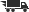Close

# Dependability for Systems With a Partitioned State Space Markov and Semi-Markov Theory and Computational Implementation

## by Attila Csenki

• ISBN: 9780387943336
• ISBN10: 0387943331

# Dependability for Systems With a Partitioned State Space Markov and Semi-Markov Theory and Computational Implementation

## by Attila Csenki

• List Price: \$109.00
• Binding: Paperback
• Publisher: Springer Verlag
• Publish date: 08/01/1994
• ISBN: 9780387943336
• ISBN10: 0387943331
used \$7.28
You save: 93%
new \$96.53
You save: 11%
FREE economy shipping!
Description: 1 Stochastic processes for dependability assessment.- 1.1 Markov and semi-Markov processes for dependability assessment.- 1.2 Example systems.- 1.2.1 Markov models.- 1.2.2 Semi-Markov models.- 2 Sojourn times for discrete-parameter Markov chains.- 2.1 Distribution theory for sojourn times and related variables.- 2.1.1 Key results: sojourn times in a subset of the state space.- 2.1.2 Distribution theory for variables related to the sojourn time vector.- 2.1.3 The joint distribution of sojourn times in A1 and A2 by the generalised renewal argument.- 2.1.4 Tabular summary of results about sojourn times and related variables.- 2.2 An application: the sequence of repair events for a three-unit power transmission model.- 2.2.1 The number of major repair events Rn in a repair sequence of lenght n.- 2.2.2 Numerical results.- 2.2.3 Implementation with MATLAB.- 2.2.4 MATLAB code.- 3 The number of visits until absorption to subsets of the state space by a discrete-parameter Markov chain: the multivariate case.- 3.1 The probability generating function of M and the probability mass function of L.- 3.2 Further results for n ? {2, 3}.- 3.3 Tabular summary of results in Sections 3.1 and 3.2.- 3.4 A power transmission reliabilty application.- 3.4.1 Numerical results.- 3.4.2 MATLAB code.- 4 Sojourn times for continuous-parameter Markov chains.- 4.1 Distribution theory for sojourn times.- 4.2 Some further distribution results related to sojourn times.- 4.3 Tabular summary of results in Sections 4.1 and 4.2.- 4.4 An application: further dependability characteristics of the three-unit power transmission model.- 4.4.1 Numerical results.- 4.4.2 MATLAB code.- 5 The number of visits to a subset of the state space by a continuous-parameter irreducible Markov chain during a finite time interval.- 5.1 The variable \$\${M_{{A_1}}}(t)\$\$.- 5.1.1 The main result.- 5.1.2 The proof of Theorem 5.1.- 5.2 An application: the number of repairs of a two-unit power transmission system during a finite time interval.- 5.2.1 Numerical results and implementation issues.- 5.2.2 MATLAB code.- 6 A compound measure of dependability for continuous-time Markov models of repairable systems.- 6.1 The dependability measure and its evaluation by randomization.- 6.2 The evaluation of ?(k, i, n).- 6.2.1 The auxiliary absorbing Markov chain X(k).- 6.2.2 The closed form expression for ?(k, i, n).- 6.3 Application and computational experience.- 6.3.1 Computational implementation.- 6.3.2 Application: two parallel units with a single repairman.- 6.3.3 Implementation in MATLAB.- 6.3.4 MATLAB code.- 7 A compound measure of dependability for continuous-time absorbing Markov systems.- 7.1 The dependability measure.- 7.2 Proof of Theorem 7.1.- 7.2.1 Proof outline.- 7.2.2 An auxiliary result.- 7.2.3 Proof details.- 7.3 Application: the Markov model of the three-unit power transmission system revisited.- 8 Sojourn times for finite semi-Markov processes.- 8.1 A recurrence relation for the Laplace transform of the vector of sojourn times.- 8.2 Laplace transforms of vectors of sojourn times.- 8.2.1 S is partitioned into three subsets (n = 2).- 8.2.2 S is partitioned into four subsets (n = 3).- 8.3 Proof of Theorem 8.1.- 9 The number of visits to a subset of the state space by an irreducible semi-Markov process during a finite time interval: moment results.- 9.1 Preliminaries on the moments of \$\${M_{{A_1}}}(t)\$\$.- 9.2 Main result: the Laplace transform of the measures U'.- 9.3 Proof of Theorem 9.2.- 9.4 Reliability applications.- 9.4.1 The alternating renewal process.- 9.4.2 Two units in parallel with an arbitrary change out time distribution.- 10 The number of visits to a subset of the state space by an irreducibe semi-Markov process during a finite time interval: the probability mass function.- 10.1 The Laplace transform of the probability mass function of \$\${M_{{A_1}}}(t)\$\$.- 10.1.1 A recurrence relation in the Laplace transform domain.- 10.1.2 The direct computation of Laplace transforms.- 10.2 Numerical inversion of Laplace transforms using Laguerre polynomials and fast Fourier transform.- 10.2.1 A summary of the numerical Laplace transform inversion scheme.- 10.2.2 The inversion scheme in the NAG implementation.- 10.3 Reliability applications.- 10.3.1 The Markov model of the two-unit power transmission system revisited.- 10.3.2 The two-unit semi-Markov model revisited.- 10.4 Implementation issues.- 10.4.1 The NAG library.- 10.4.2 Input data file and FORTRAN 77 code for the Markov model.- 10.4.3 MATLAB implementation of the Laplace transform inversion algorithm.- 10.4.4 MATLAB code.- 11 The number of specific service levels of a repairable semi-Markov system during a finite time interval: joint distributions.- 11.1 A recurrence relation for h(t; m1, m2) in the Laplace transform domain.- 11.2 A computation scheme for the Laplace transforms.- 12 Finite time-horizon sojourn times for finite semi-Markov processes.- 12.1 The double Laplace transform of finite-horizon sojourn times.- 12.2 An application: the alternating renewal process.- 12.2.1 Laplace transforms.- 12.2.2 Symbolic inversion with MAPLE and computational experience.- 12.2.3 MAPLE code.- Postscript.- References.
Expand description
 Seller: Metakomet Books ★★★★★ ★★★★★ Location: Concord, MA Condition: Very Good8vo-over 7Â¾"-9Â¾" tall. Touch fading at spine. No. 1025 written at top,[...] 8vo-over 7Â¾"-9Â¾" tall. Touch fading at spine. No. 1025 written at top, inside front cover. Bit of light edge toning. Otherwise, very clean and sound. Lecture Notes in Statistics, 90. Price: \$61.87 Comments: 8vo-over 7Â¾"-9Â¾" tall. Touch fading at spine. No. 1025 written at top,[...] 8vo-over 7Â¾"-9Â¾" tall. Touch fading at spine. No. 1025 written at top, inside front cover. Bit of light edge toning. Otherwise, very clean and sound. Lecture Notes in Statistics, 90.